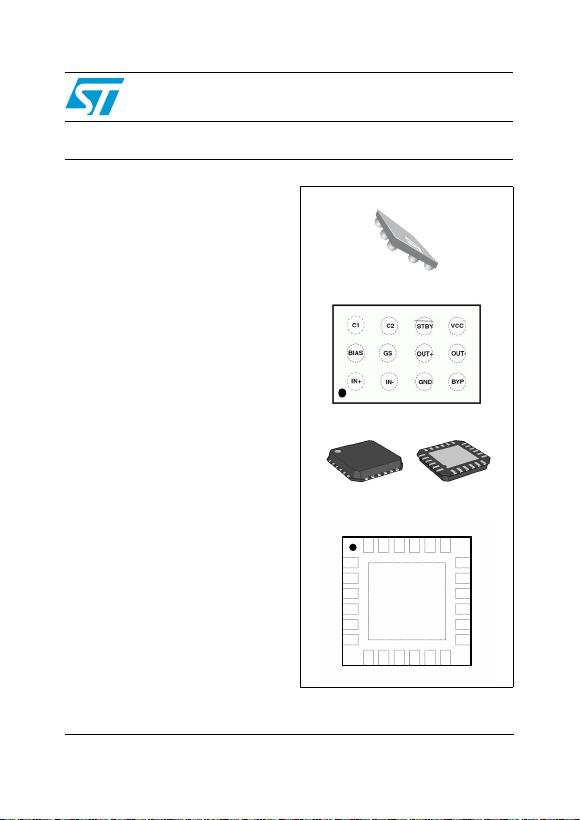August 2009 Doc ID 11015 Rev 6 1/25
25
TS472
Very low noise microphone preamplifier with
2.0 V bias output and active low standby mode
Features
Low noise: 10 nV/Hz typ. equivalent input
noise at F = 1 kHz
Fully-differential input/output
2.2 to 5.5 V single supply operation
Low power consumption at 20 dB: 1.8 mA
Fast start up time at 0 dB: 5 ms typ.
Low distortion: 0.1% typ.
40 kHz bandwidth regardless of the gain
Active low standby mode function (1 μA max)
Low noise 2.0 V microphone bias output
Available in flip-chip lead-free package and in
QFN24 4 x 4 mm package
ESD protection (2 kV)
Applications
Video and photo cameras with sound input
Sound acquisition and voice recognition
Video conference systems
Notebook computers and PDAs
Description
The TS472 is a differential-input microphone
preamplifier optimized for high-performance PDA
and notebook audio systems.
This device features an adjustable gain from 0 to
40 dB with excellent power-supply and common-
mode rejection ratios. In addition, the TS472 has
a very low noise microphone bias generator of
2V.
It also includes a complete shutdown function,
with active low standby mode.
Flip-chip - 12 bumps
Pin connections (top view)
QFN24
Pin connections (top view)
1
2
3
4
5
6
7
13
14
15
16
17
18
192021222324
NC
BYP
NC
NC NC NC
NC
NC
NCNCNC
GND
IN-
8 9 10 11 12
NC
IN+ GS BIAS
C1
C2
OUT-
OUT+
GND STBY VCC
www.st.comContents TS472
2/25 Doc ID 11015 Rev 6
Contents
1 Typical application schematic . . . . . . . . . . . . . . . . . . . . . . . . . . . . . . . . . 3
2 Absolute maximum ratings . . . . . . . . . . . . . . . . . . . . . . . . . . . . . . . . . . . 5
3 Electrical characteristics . . . . . . . . . . . . . . . . . . . . . . . . . . . . . . . . . . . . . 6
4 Application information . . . . . . . . . . . . . . . . . . . . . . . . . . . . . . . . . . . . . 13
4.1 Differential configuration principle . . . . . . . . . . . . . . . . . . . . . . . . . . . . . . 13
4.2 Higher cut-off frequency . . . . . . . . . . . . . . . . . . . . . . . . . . . . . . . . . . . . . . 13
4.3 Lower cut-off frequency . . . . . . . . . . . . . . . . . . . . . . . . . . . . . . . . . . . . . . 14
4.4 Low-noise microphone bias source . . . . . . . . . . . . . . . . . . . . . . . . . . . . . 14
4.5 Gain settings . . . . . . . . . . . . . . . . . . . . . . . . . . . . . . . . . . . . . . . . . . . . . . 15
4.6 Wake-up time . . . . . . . . . . . . . . . . . . . . . . . . . . . . . . . . . . . . . . . . . . . . . . 16
4.7 Standby mode . . . . . . . . . . . . . . . . . . . . . . . . . . . . . . . . . . . . . . . . . . . . . 17
4.8 Layout considerations . . . . . . . . . . . . . . . . . . . . . . . . . . . . . . . . . . . . . . . . 17
4.9 Single-ended input configuration . . . . . . . . . . . . . . . . . . . . . . . . . . . . . . . 17
4.10 Demonstration board . . . . . . . . . . . . . . . . . . . . . . . . . . . . . . . . . . . . . . . . 18
5 Package information . . . . . . . . . . . . . . . . . . . . . . . . . . . . . . . . . . . . . . . . 19
5.1 Flip-chip package information . . . . . . . . . . . . . . . . . . . . . . . . . . . . . . . . . 19
5.2 QFN24 package information . . . . . . . . . . . . . . . . . . . . . . . . . . . . . . . . . . 21
6 Ordering information . . . . . . . . . . . . . . . . . . . . . . . . . . . . . . . . . . . . . . . 23
7 Revision history . . . . . . . . . . . . . . . . . . . . . . . . . . . . . . . . . . . . . . . . . . . 24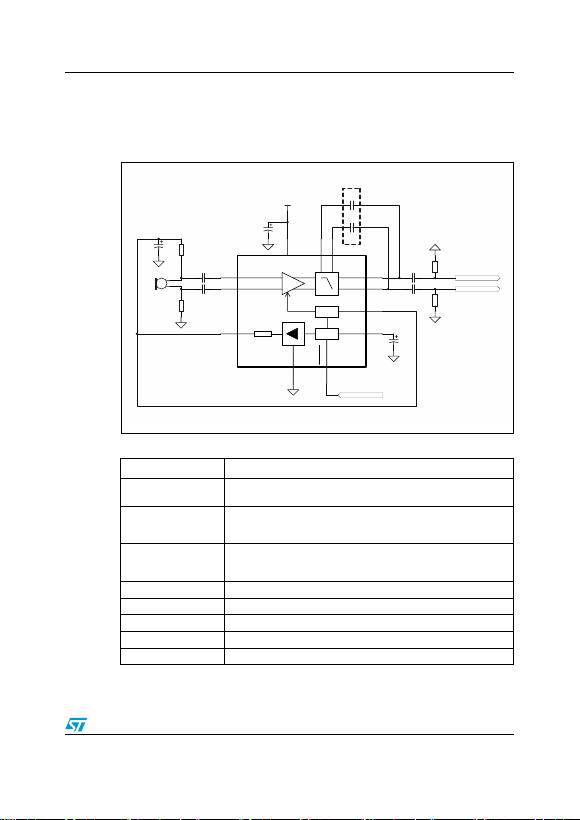TS472 Typical application schematic
Doc ID 11015 Rev 6 3/25
1 Typical application schematic
Figure 1 shows a typical application schematic for the TS472.
Figure 1. Application schematic (flip-chip)
Table 1. Description of external components
Components Functional description
Cin+, Cin-
Input coupling capacitors that block the DC voltage at the amplifier input
terminal.
Cout+, Cout-
Output coupling capacitors that block the DC voltage coming from the
amplifier output terminal (pins C2 and D2) and determine the lower cut-off
frequency (see Section 4.3: Lower cut-off frequency).
Rout+, Rout-
Output load resistors used to charge the output coupling capacitors Cout.
These output resistors can be represented by an input impedance of a
following stage.
Rpos, Rneg Polarizing resistors for biasing of a microphone.
CsSupply bypass capacitor that provides power supply filtering.
CbBypass pin capacitor that provides half-supply filtering.
C1, C2Low pass filter capacitors allowing to cut the high frequency.
C3Bias output filtering capacitor.
VCC
Electret Mic
Rpos
Rneg
Standby Control
Positive Output
Negative Output
1uF
Cb
1uF
Cs
1uF
C3
C1
C2
Cin+
Cin-
+
Cout+
Cout-
Rout+
Rout-
Bias
STDBY
IN-
IN+
G
C1
C2
OUT+
OUT-
GAIN
BYPASS
BIAS
GND
Vcc
SELECT
2.0V
U1 TS472_FC
Optional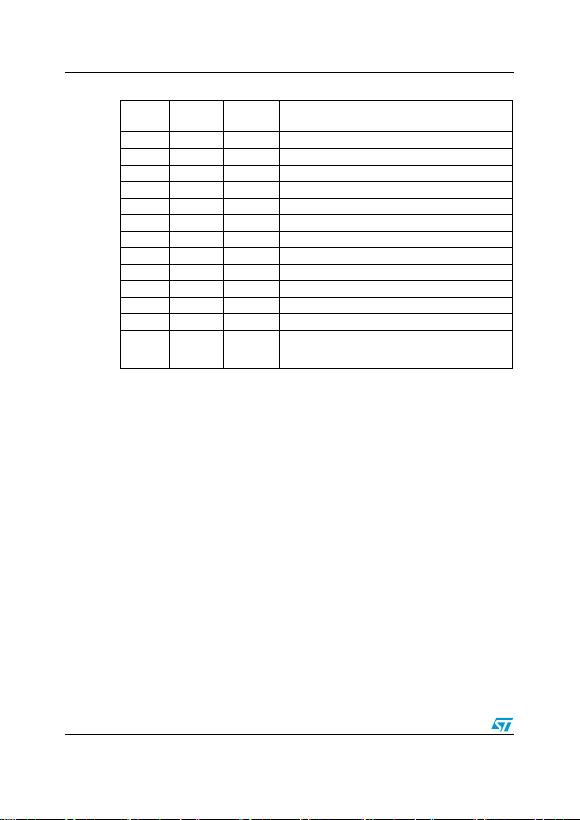Typical application schematic TS472
4/25 Doc ID 11015 Rev 6
Table 2. Pin descriptions
Pin name Flip-chip
designator
QFN
designator Pin description
IN+ A1 8 Positive differential input
IN- B1 5 Negative differential input
BIAS A2 10 2 V bias output
GND C1 4, 22 Ground
STBY C3 21 Standby
BYP D1 2 Bypass
GS B2 9 Gain select
OUT- D2 16 Negative differential output
OUT+ C2 17 Positive differential output
C1 A3 14 Low-pass filter capacitor
C2 B3 15 Low-pass filter capacitor
Vcc D3 20 Power supply
NC ---
3, 6, 7, 11,
12, 13, 18,
19, 23, 24
Not connected, floating pins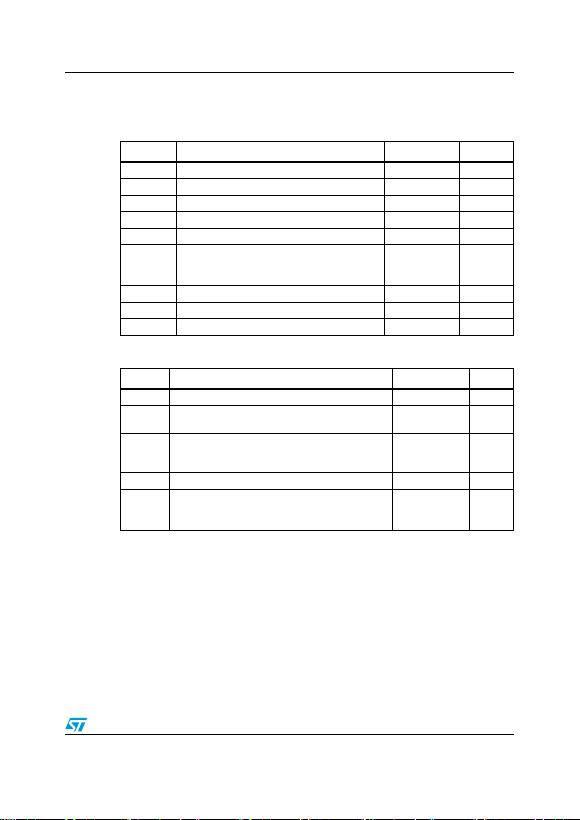TS472 Absolute maximum ratings
Doc ID 11015 Rev 6 5/25
2 Absolute maximum ratings
Table 3. Absolute maximum ratings
Symbol Parameter Value Unit
VCC Supply voltage (1)
1. All voltage values are measured with respect to the ground pin.
6V
ViInput voltage -0.3 to VCC+0.3 V
Toper Operating free air temperature range -40 to + 85 °C
Tstg Storage temperature -65 to +150 °C
TjMaximum junction temperature 150 °C
Rthja
Thermal resistance junction to ambient:
Flip-chip
QFN24
180
110 °C/W
ESD Human body model 2 kV
ESD Machine model 200 V
Lead temperature (soldering, 10sec) 250 °C
Table 4. Operating conditions
Symbol Parameter Value Unit
VCC Supply voltage 2.2 to 5.5 V
ATypical differential gain
(GS connected to 4.7 kΩ or bias)20 dB
VSTBY
Standby voltage input:
Device ON
Device OFF
1.5 VSTBY VCC
GND VSTBY 0.4
V
Top Operational free air temperature range -40 to +85 °C
Rthja
Thermal resistance junction to ambient:
Flip-chip
QFN24
150
60 °C/W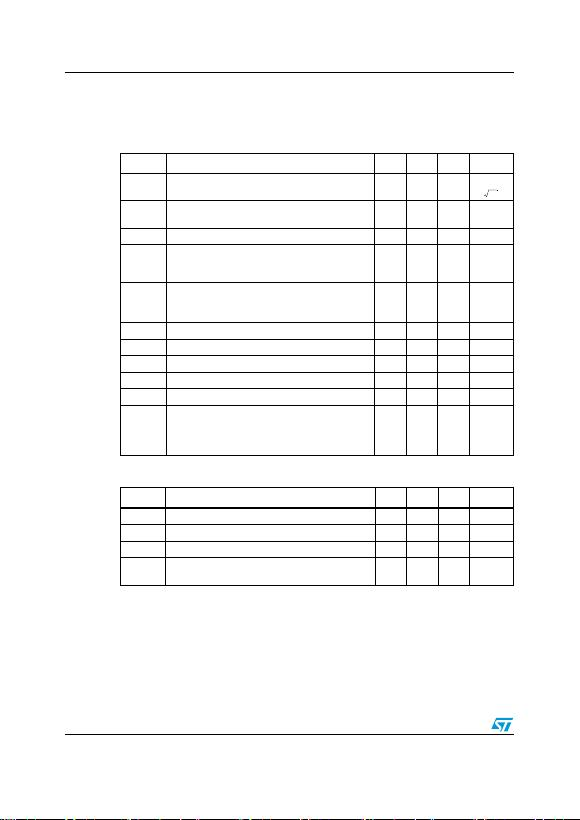Electrical characteristics TS472
6/25 Doc ID 11015 Rev 6
3 Electrical characteristics
Table 5. Electrical characteristics at VCC = 3 V with GND = 0 V, Tamb = 25° C
(unless otherwise specified)
Symbol Parameter Min. Typ. Max. Unit
en
Equivalent input noise voltage density
REQ =100Ω at 1 kHz 10
THD+N Total harmonic distortion + noise
20 Hz F 20 kHz, gain = 20 dB, Vin =50mV
RMS
0.1 %
Vin Input voltage, gain = 20 dB 10 70 mVRMS
BW
Bandwidth at -3 dB
Bandwidth at -1 dB
pin A3, B3 floating
40
20 kHz
G
Overall output voltage gain (Rgs variable):
Minimum gain, Rgs infinite
Maximum gain, Rgs = 0
-3
39.5
-1.5
41
0
42.5
dB
Zin Input impedance referred to GND 80 100 120 kΩ
ICC Supply current, gain = 20 dB 1.8 2.4 mA
ISTBY Standby current 1 μA
PSRR
Power supply rejection ratio, gain = 20 dB,
F = 217 Hz, Vripple = 200 mVpp, inputs grounded
Differential output
Single-ended outputs,
-70
-46
dB
Table 6. Bias output: VCC = 3 V, GND = 0 V, Tamb = 25° C
(unless otherwise specified)
Symbol Parameter Min. Typ. Max. Unit
Vout No load condition 1.9 2 2.1 V
Rout Output resistance 80 100 120 W
Iout Output bias current 2 mA
PSRR Power supply rejection ratio, F = 217 Hz,
Vripple = 200 mVpp 70 80 dB
nV
Hz
------------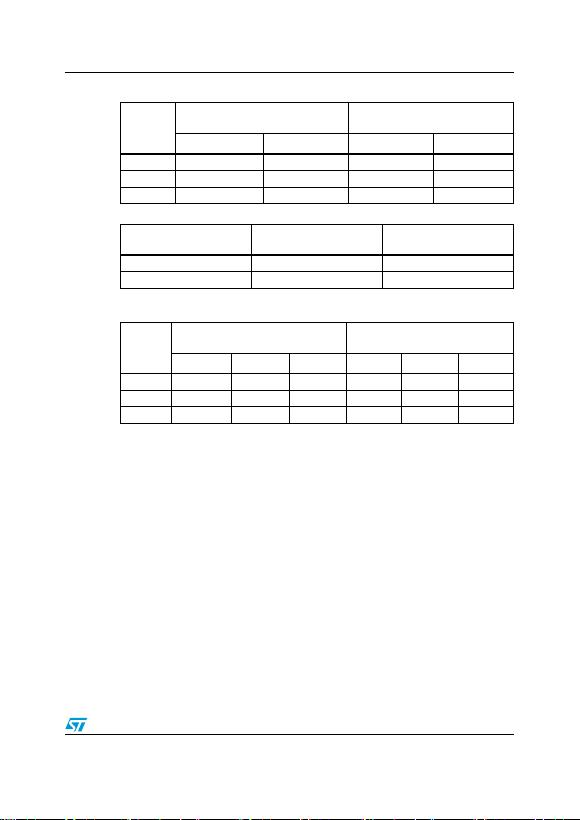TS472 Electrical characteristics
Doc ID 11015 Rev 6 7/25
Table 7. Differential RMS noise voltage
Gain
(dB)
Input referred noise voltage
(μVRMS)
Output noise voltage
(μVRMS)
Unweighted filter A-weighted filter Unweighted filter A-weighted filter
015 10 15 10
20 3.4 2.3 34 23
40 1.4 0.9 141 91
Table 8. Bias output RMS noise voltage
C3(1)
(μF)
1. Bias output filtering capacitor.
Unweighted filter
(μVRMS)
A-weighted filter
(μVRMS)
154.4
10 2.2 1.2
Table 9. SNR (signal to noise ratio), THD+N < 0.5%
Gain
(dB)
Unweighted filter 20 Hz - 20 kHz
(dB)
A-weighted filter
(dB)
VCC =2.2V V
CC =3V V
CC =5.5V V
CC =2.2V V
CC =3V V
CC =5.5V
0 75 76 76798080
20 82 83 83 89 90 90
40 70 72 74 80 82 84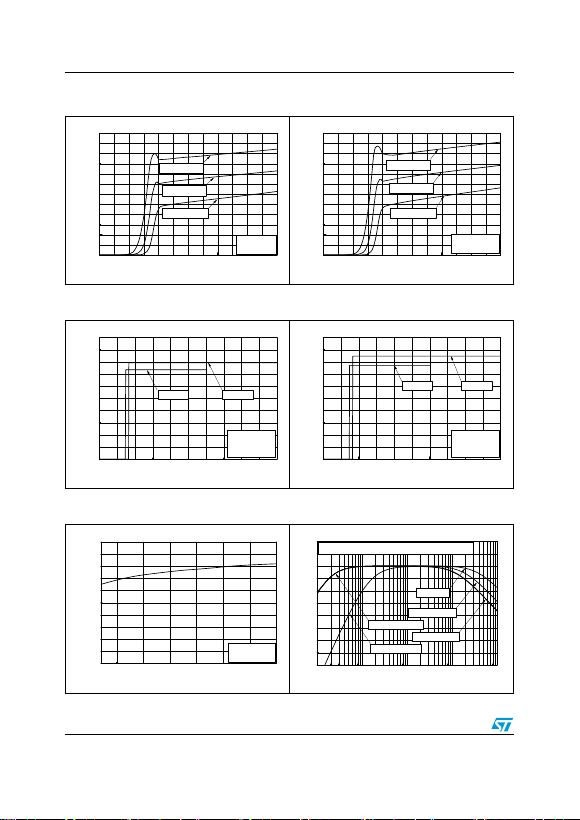Electrical characteristics TS472
8/25 Doc ID 11015 Rev 6
Figure 2. Current consumption vs. power
supply voltage
Figure 3. Current consumption vs. power
supply voltage
0123456
0.0
0.5
1.0
1.5
2.0
2.5
3.0
Tamb=85°C
Tamb=25°C
Tamb=-40°C
GS floating
Current Consumption (mA)
Power Supply Voltage (V)
0123456
0.0
0.5
1.0
1.5
2.0
2.5
3.0
Tamb=85°C
Tamb=25°C
Tamb=-40°C
GS grounded
Current Consumption (mA)
Power Supply Voltage (V)
Figure 4. Current consumption vs. standby
voltage
Figure 5. Current consumption vs. standby
voltage
012345
0.0
0.5
1.0
1.5
2.0
2.5
Vcc=5V
Vcc=3V
GS floating
Tamb = 25°C
Current Consumption (mA)
Standby Voltage (V)
012345
0.0
0.5
1.0
1.5
2.0
2.5
Vcc=5V
Vcc=3V
GS grounded
Tamb = 25°C
Current Consumption (mA)
Standby Voltage (V)
Figure 6. Standby threshold voltage vs.
power supply voltage
Figure 7. Frequency response
345
0.0
0.2
0.4
0.6
0.8
1.0
Tamb = 25°C
Standby Treshold Voltage (V)
Power Supply Voltage (V)
2.2 5.5
10 100 1000 10000 100000
-20
-10
0
10
20
30
C1,C2=220pF
C1,C2=100pF
Cin,Cout=10nF
Cin,Cout=100nF
no C1,C2
Cb=1
μ
F, T
AMB
=25
°
C, Gain=20dB, Rout=100k
Ω
PSRR (dB)
Frequency (Hz)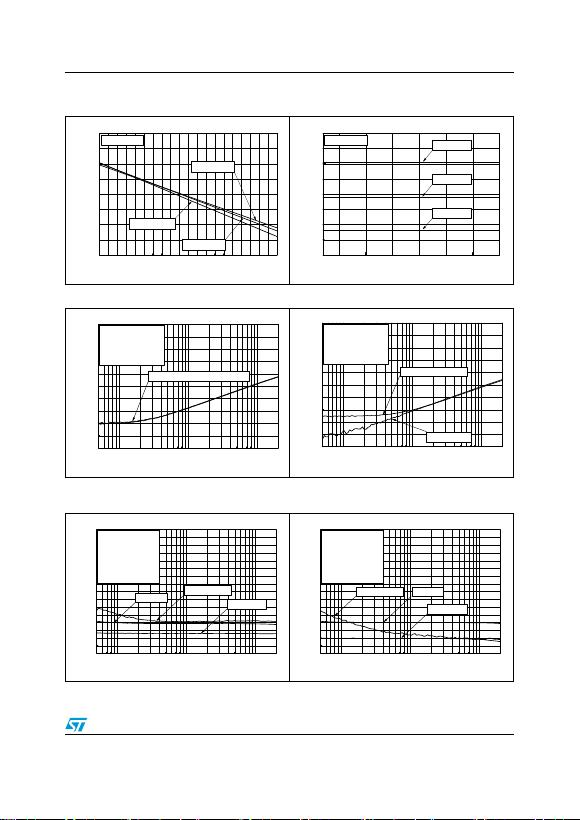TS472 Electrical characteristics
Doc ID 11015 Rev 6 9/25
Figure 8. Bias output voltage vs. bias output
current
Figure 9. Bias output voltage vs. power
supply voltage
01234
1.4
1.6
1.8
2.0
2.2
Tamb=85°C
Tamb=25°C
Bias Output Voltage (V)
Bias Output Current (mA)
Tamb=-40°C
Vcc=2.5-6V
345
1.4
1.6
1.8
2.0
2.2
Ibias=4mA
Ibias=2mA
Tamb=25°C Ibias=0mA
Bias Output Voltage (V)
Power Supply Voltage (V)
2.2 5.5
Figure 10. Bias PSRR vs. frequency Figure 11. Bias PSRR vs. frequency
100 1000 10000
-100
-80
-60
-40
-20
0
Vripple=200mVpp
Vcc=3V
Cb=1
μ
F
Tamb =25
°
C
PSRR (dB)
Frequency (Hz)
50 20k
Bias floating or 1k
Ω
to GND
100 1000 10000
-100
-80
-60
-40
-20
0
Bias = 1k
Ω
to GND
Vripple=200mVpp
Vcc=5V
Cb=1
μ
F
Tamb=25
°
C
PSRR (dB)
Frequency (Hz)
50 20k
Bias floating
Figure 12. Differential output PSRR vs.
frequency
Figure 13. Differential output PSRR vs.
frequency
100 1000 10000
-80
-70
-60
-50
-40
-30
-20
-10
0
GS=bias
GS grounded
Vripple=200mVpp
Inputs grounded
Vcc=3V
Cb=1
μ
F
Cin=100nF
Tamb=25
°
C
GS floating
PSRR (dB)
Frequency (Hz)
50 20k
100 1000 10000
-80
-70
-60
-50
-40
-30
-20
-10
0
GS=bias
GS grounded
Vripple=200mVpp
Inputs grounded
Vcc=5V
Cb=1
μ
F
Cin=100nF
Tamb=25
°
C
GS floating
PSRR (dB)
Frequency (Hz)
50 20k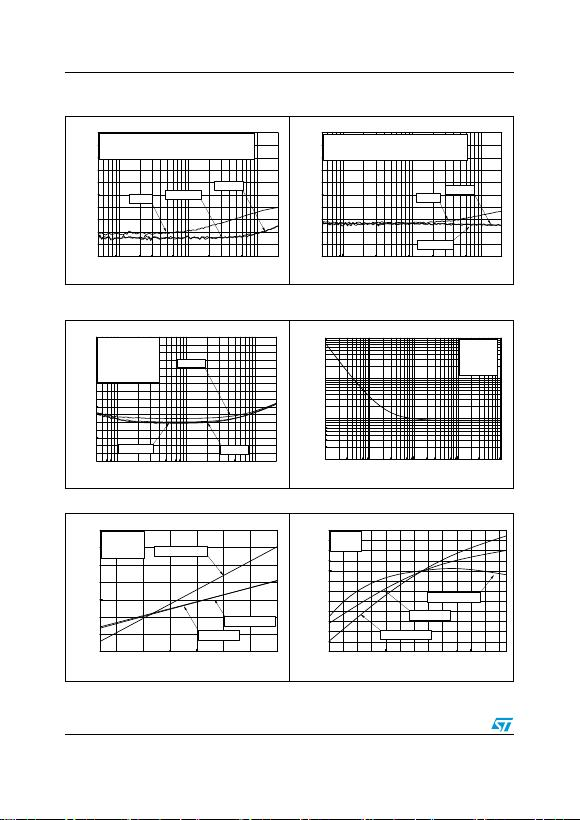Electrical characteristics TS472
10/25 Doc ID 11015 Rev 6
Figure 14. Differential output PSRR vs.
frequency
Figure 15. Differential output PSRR vs.
frequency
100 1k 10k
-100
-80
-60
-40
-20
0
V
RIPPLE
=200mV
PP
, Inputs grounded
V
CC
=3V, Minimum Gain, Cin=1
μ
F, T
AMB
=25
°
C
Cb=1
μ
F
No Cb Cb=100nF
PSRR (dB)
Frequency (Hz)
50 20k
100 1k 10k
-100
-80
-60
-40
-20
0
V
RIPPLE
=200mV
PP
, Inputs grounded
V
CC
=3V, Gain=20dB, Cin=1
μ
F, T
AMB
=25
°
C
Cb=1
μ
F
No Cb
Cb=100nF
PSRR (dB)
Frequency (Hz)
50 20k
Figure 16. Single-ended output PSRR vs.
frequency
Figure 17. Equivalent input noise voltage
density
100 1000 10000
-80
-70
-60
-50
-40
-30
-20
-10
0
Vcc=5V
Vcc=2.2V
Vcc=3V
Vripple=200mVpp
Inputs grounded
Cb=1
μ
F
Cin=100nF
Tamb=25
°
C
PSRR (dB)
Frequency (Hz)
50 20k
10 100 1k 10k 100k
1
10
100
1000
Cin=100nF
R
EQ
=100
Ω
T
AMB
=25
°
C
e
n
(
nV/
Hz
)
Frequency (Hz)
Figure 18. Δgain vs. power supply voltage Figure 19. Δgain vs. ambient temperature
345
-0.4
-0.2
0.0
0.2
0.4
0.6
0.8
1.0
Maximum Gain
Gain=20dB
F=1kHz
Vin=5mV
Tamb=25°C
Minimum Gain
Δ
Gain (dB)
Power Supply Voltage (V)
2.2 5.5
-40-200 20406080
-1.00
-0.75
-0.50
-0.25
0.00
0.25
0.50
Maximum Gain
F=1kHz
V
IN
=5mV
Gain=20dB
Minimum Gain
Δ
Gain (dB)
Ambient Temperature (°C)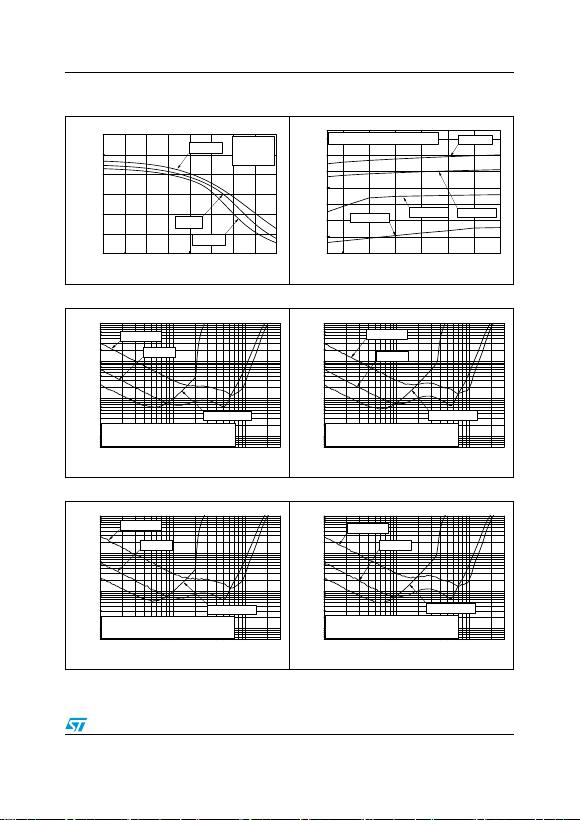TS472 Electrical characteristics
Doc ID 11015 Rev 6 11/25
Figure 20. Maximum input voltage vs. gain,
THD+N<1%
Figure 21. Maximum input voltage vs. power
supply voltage, THD+N<1%
0 10203040
0
50
100
150
V
CC
=2.2V
T
AMB
=25°C
F=1kHz
THD+N<1%
Maximum Input Voltage
(
mV
RMS
)
Gain (dB)
V
CC
=5.5V
V
CC
=3V
345
0
20
40
60
80
100
120
140
Gain=20dB
Gain=0dB
T
AMB
=25°C, F=1kHz, THD+N<1%
Maximum Input Voltage (mVRMS)
Power Supply Voltage (V)
Gain=30dB
Gain=40dB
2.2 5.5
Figure 22. THD+N vs. input voltage Figure 23. THD+N vs. input voltage
1E-3 0.01 0.1
0.01
0.1
1
10
GS=bias
GS grounded
Tamb=25°C, Vcc=3V, F=100Hz,
Cb=1
μ
F, RL=10k
Ω
, BW=100Hz-120kHz
THD+N (%)
Input Voltage (V)
0.3
GS floating
1E-3 0.01 0.1
0.01
0.1
1
10
GS grounded
GS floating
GS=bias
Tamb=25°C, Vcc=5V, F=100Hz,
Cb=1
μ
F, RL=10k
Ω
, BW=100Hz-120kHz
THD+N (%)
Input Voltage (V)
0.3
Figure 24. THD+N vs. input voltage Figure 25. THD+N vs. input voltage
1E-3 0.01 0.1
0.01
0.1
1
10
GS grounded
GS=bias
Tamb=25°C, Vcc=3V, F=1kHz,
Cb=1
μ
F, RL=10k
Ω
, BW=100Hz-120kHz
THD+N (%)
Input Voltage (V)
0.3
GS floating
1E-3 0.01 0.1
0.01
0.1
1
10
GS grounded
GS=bias
GS floating
Tamb=25°C, Vcc=5V, F=1kHz,
Cb=1
μ
F, RL=10k
Ω
, BW=100Hz-120kHz
THD+N (%)
Input Voltage (V)
0.3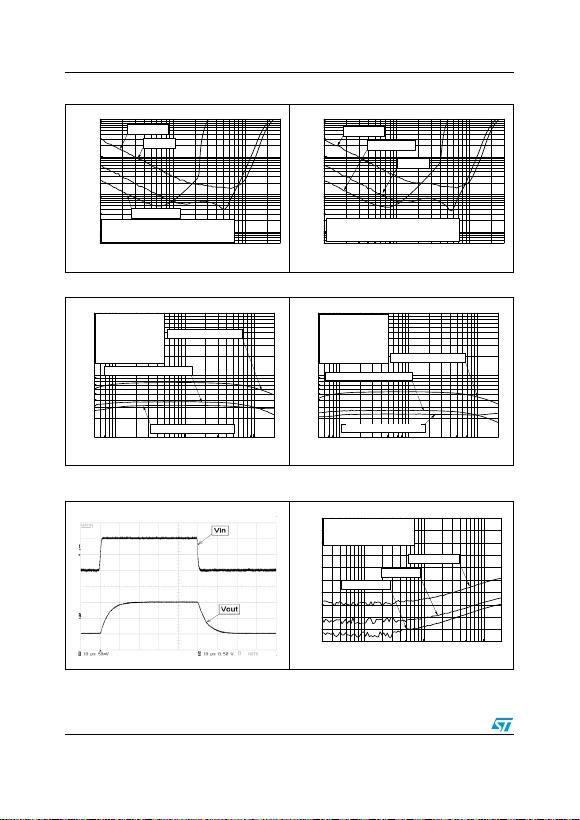Electrical characteristics TS472
12/25 Doc ID 11015 Rev 6
Figure 26. THD+N vs. input voltage Figure 27. THD+N vs. input voltage
1E-3 0.01 0.1
0.01
0.1
1
10
GS grounded
GS=bias
Tamb=25°C, Vcc=3V, F=20kHz,
Cb=1
μ
F, RL=10k
Ω
, BW=100Hz-120kHz
THD+N (%)
Input Voltage (V)
0.3
GS floating
1E-3 0.01 0.1
0.01
0.1
1
10
GS floating
GS=bias
GS grounded
Tamb=25°C, Vcc=5V, F=20kHz,
Cb=1
μ
F, RL=10k
Ω
, BW=100Hz-120kHz
THD+N (%)
Input Voltage (V)
0.3
Figure 28. THD+N vs. frequency Figure 29. THD+N vs. frequency
100 1000 10000
0.1
1
10
GS=bias, Vin=100mV
GS floating, Vin=100mV
GS grounded, Vin=20mV
Tamb=25°C
Vcc=3V
RL=10k
Ω
Cb=1
μ
F
BW=100Hz-120kHz
20k50
THD + N (%)
Frequency (Hz)
100 1000 10000
0.1
1
10
GS=bias, Vin=100mV
GS floating, Vin=100mV
GS grounded, Vin=20mV
Tamb=25
°
C
Vcc=5V
RL=10k
Ω
Cb=1
μ
F
BW=100Hz-120kHz
20k50
THD + N (%)
Frequency (Hz)
Figure 30. Transient response Figure 31. Common mode rejection ratio
(CMRR) vs frequency
100 1k 10k
-100
-80
-60
-40
-20
0
Δ
Vicm=200mVpp, V
CC
=3V
C
IN
=1
μ
F, T
AMB
=25°C
Gain=20dB
Maximum Gain
Minimum Gain
CMRR (dB)
Frequency (Hz)
20 20k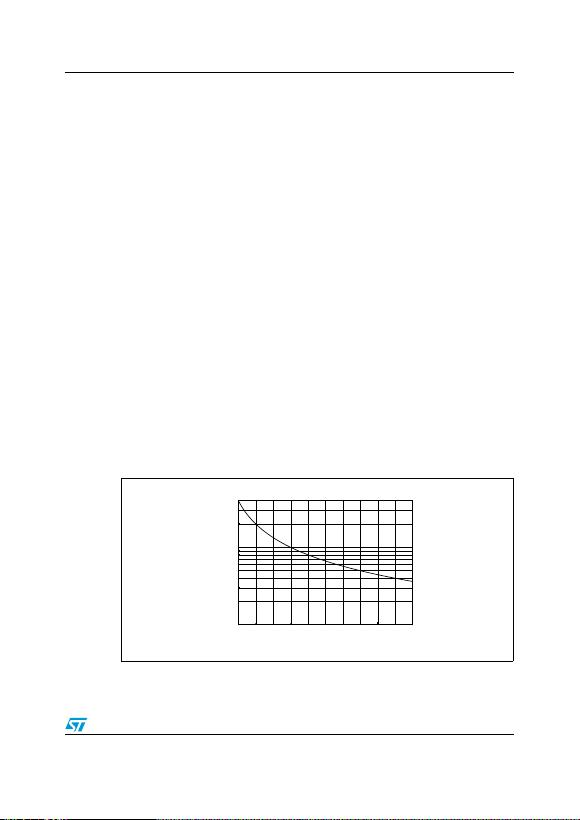TS472 Application information
Doc ID 11015 Rev 6 13/25
4 Application information
4.1 Differential configuration principle
The TS472 is a fully-differential input/output microphone preamplifier. The TS472 also
includes a common-mode feedback loop that controls the output bias value to average it at
VCC/2. This allows the device to always have a maximum output voltage swing, and by
consequence, maximize the input dynamic voltage range.
The advantages of a fully-differential amplifier are:
Very high PSRR (power supply rejection ratio).
High common mode noise rejection.
In theory, the filtering of the internal bias by an external bypass capacitor is not
necessary. However, to reach maximum performance in all tolerance situations, it is
better to keep this option.
4.2 Higher cut-off frequency
The higher cut-off frequency FCH of the microphone preamplifier depends on the external
capacitors C1, C2.
TS472 has an internal first order low-pass filter (R = 40 kΩ, C = 100 pF) to limit the highest
cut-off frequency on 40 kHz (with a 3 dB attenuation). By connecting C1, C2 you can
decrease FCH by applying the following formula.
Figure 32 represents the higher cut-off frequency in Hz versus the value of the output
capacitors C1, C2 in nF.
Figure 32. Higher cut-off frequency vs. output capacitors
For example, FCH is almost 20 kHz with C1,2 =100 pF.
FCH
1
2π40 3
×10 C12,100 12
×10+()⋅⋅
----------------------------------------------------------------------------------------------=
200 400 600 800 1000
1
10
Higher Cut-off Frequency (kHz)
C1, C2 (pF)
40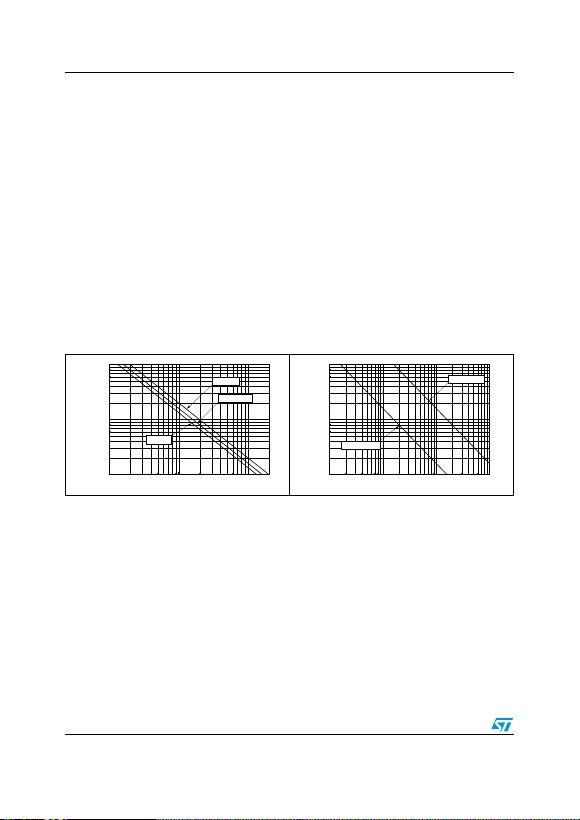Application information TS472
14/25 Doc ID 11015 Rev 6
4.3 Lower cut-off frequency
The lower cut-off frequency FCL of the microphone preamplifier depends on the input
capacitors Cin and output capacitors Cout. These input and output capacitors are mandatory
in an application because of DC voltage blocking.
The input capacitors Cin in series with the input impedance of the TS472 (100 kΩ) are
equivalent to a first order high-pass filter. Assuming that FCL is the lowest frequency to be
amplified (with a 3 dB attenuation), the minimum value of Cin is:
The capacitors Cout in series with the output resistors Rout (or an input impedance of the
next stage) are also equivalent to a first order high-pass filter. Assuming that FCL is the
lowest frequency to be amplified (with a 3 dB attenuation), the minimum value of Cout is:
Figure 33 and Figure 34 give directly the lower cut-off frequency (with 3 dB attenuation)
versus the value of the input or output capacitors.
Note: If FCL is kept the same for calculation purposes, take into account that the 1st order high-
pass filter on the input and the 1st order high-pass filter on the output create a 2nd order
high-pass filter in the audio signal path with an attenuation of 6 dB on FCL and a roll-off of
4.4 Low-noise microphone bias source
The TS472 provides a very low noise voltage and power supply rejection BIAS source
designed for biasing an electret condenser microphone cartridge. The BIAS output is
typically set at 2.0 VDC (no load conditions), and can typically source 2 mA with respect to
drop-out, determined by the internal 100 Ω resistance (for detailed load regulation curves
see Figure 8).
Cin
1
2πFCL 100 3
×10⋅⋅
------------------------------------------------------=
Cout
1
2πFCL Rout
⋅⋅
-------------------------------------------=
Figure 33. Lower cut-off frequency vs. input
capacitors
Figure 34. Lower cut-off frequency vs. output
capacitors
1 10 100
10
100
1000
Typical Zin
ZinMAX
Lower Cut-off frequency (Hz)
Cin (nF)
ZinMIN
1 10 100 1000
10
100
1000
Rout=10k
Ω
Lower Cut-off frequency (Hz)
Cout (nF)
Rout=100k
Ω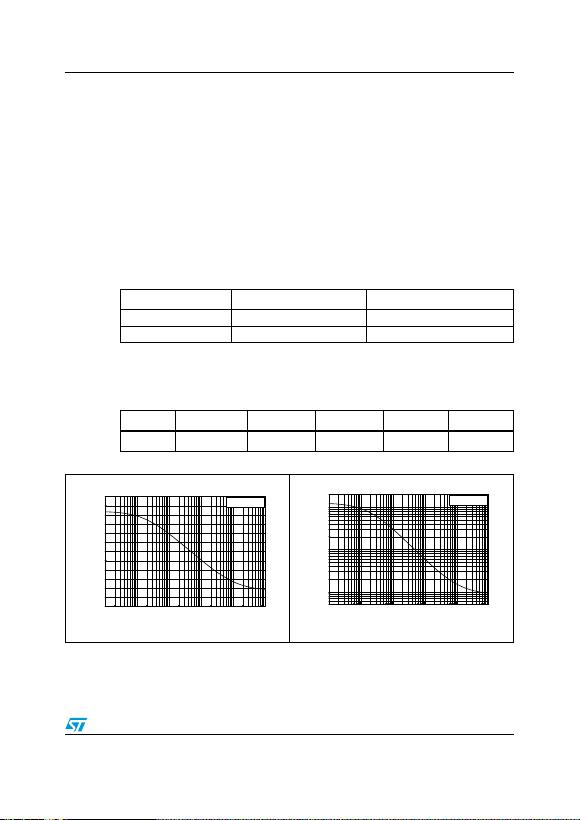TS472 Application information
Doc ID 11015 Rev 6 15/25
4.5 Gain settings
The gain in the application depends mainly on:
the sensitivity of the microphone,
the distance to the microphone,
the audio level of the sound,
the desired output level.
The sensitivity of the microphone is generally expressed in dB/Pa, referenced to 1 V/Pa. For
example, the microphone used in testing had an output voltage of 6.3 mV for a sound
pressure of 1 Pa (where Pa is the pressure unit, Pascal). Expressed in dB, the sensitivity is:
20Log(0.0063) = -44 dB/Pa
To facilitate the first approach, Ta b l e 1 0 gives voltages and gains used with a low-cost omni-
directional electret condenser microphone of -44 dB/Pa.
The gain of the TS472 microphone preamplifier can be set as follows.
1. From -1.5 dB to 41 dB by connecting an external grounded resistor RGS to the GS pin.
This enables the gain to be adapted more precisely to each application.
2. To 20 dB by applying VGS > 1VDC on the gain select (GS) pin. This setting can help to
reduce a number of external components in an application, because 2.0 VDC is
provided by the TS472 itself on the BIAS pin.
Table 10. Typical TS472 gain vs. distance to the microphone (sensitivity -44 dB/Pa)
Distance to microphone Microphone output voltage TS472 gain
1cm 30mV
RMS 20
20 cm 3 mVRMS 100
Table 11. Selected gain vs. gain select resistor
Gain (dB) 010203040
RGS (Ω)470k 27k 4k7 1k 68
Figure 35. Gain in dB vs. gain select resistor Figure 36. Gain in V/V vs. gain select resistor
10 100 1k 10k 100k 1M
-10
0
10
20
30
40
50
Tamb=25
°
C
Gain (dB)
R
GS
(
Ω
)
10 100 1k 10k 100k 1M
1
10
100
Gain (V/V)
Tamb=25
°
C
R
GS
(
Ω
)